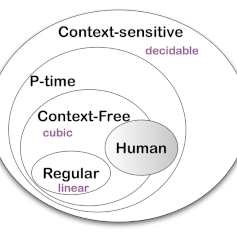# Theory of Formal Languages

## Pre-requisites, Course Objectives and outcomes

### Course Objectives

The objective the Theory of Formal languages course is to understand the theory of formal languages beyond the basic theory of computation taught as an undergraduate course in CS. The course includes: Turing machine and its variants, complexity of computation on these variants, and universal Turing machine.

Yet, other objective is, to study functions: computable functions, primitive recursive functions, partial functions, computable predicates, and semi-computable and non-computable functions.

The other objective is, to study the decidability and computability, decidable and undecidable languages, recursive and recursively enumerable languages, and Lambda calculus.

### Outcomes

Having studied this course the scholar will understand the difference between and nature of problems in the class of computable, partially computable, and non-computable.

He/she will understand the theory of functions, tractable and intractable, and the class of functions.

The course is backbone of higher studies and research in many fields of computer science, that are related to cryptography, complexity theory, and using formal approach of for program representation and analysis.

The student will learn Lambda calculus and its applications.

## Turing machine and its variants

The Turing machine is backbone of theory of computation. The major topics covered are Turing machines, 2-tape, 3-tape and k-tape Turing machines, and Universal Turing machines

## Linear bounded automata and context sensitive languages

The conventional computers, due to their finite memory size, are more close to linear bounded automata rather than the Turing machines.

The lecture notes presents different theorem proof and details the context-sensitive languages and theory.

## Computable Functions

The lecture notes defines the comptability, computable and Turing computable functions.

Sequential operations of Turing machines is defined to compute complex things making use of smaller functions.

## Decidability and Undecidability

The decision problems are those whose solution is in the form of Yes/No. These are called decision problems. The decision problems are also called Recursive (R) problems; thus there is a duality between decision problems and recursive problems.

The other problems types are Recursively enumerable (RE) problems. The Turing machines corresponding to these problems may give output Yes and halt, or they may continue forever.

The RE problems are also called Turing Recognizable problems.

The corresponding languages, every element is an instance of (M, w) where M is machine that accepts string w, is R or RE language

## Lambda Calculus

Lambda calculus is universal language to express computation.

Lambda calculus was developed by Alongo Church.

Turing machine, Lambda calculus, and Primitive Recursive (PR)) functions are equivalent.